Courses

# Test: Cartesian Equation Of A Line

## 10 Questions MCQ Test Mathematics (Maths) Class 12 | Test: Cartesian Equation Of A Line

Description
This mock test of Test: Cartesian Equation Of A Line for JEE helps you for every JEE entrance exam. This contains 10 Multiple Choice Questions for JEE Test: Cartesian Equation Of A Line (mcq) to study with solutions a complete question bank. The solved questions answers in this Test: Cartesian Equation Of A Line quiz give you a good mix of easy questions and tough questions. JEE students definitely take this Test: Cartesian Equation Of A Line exercise for a better result in the exam. You can find other Test: Cartesian Equation Of A Line extra questions, long questions & short questions for JEE on EduRev as well by searching above.
QUESTION: 1

Solution:
QUESTION: 2

Solution:
QUESTION: 3

### The Cartesian equation of the line which passes through the point (2, -2, -1) and parallel to the line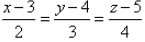, is given by

Solution:
QUESTION: 4

If the vector equation of a line is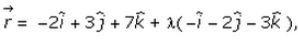find its caryesian equation.

Solution:

r = -2i + 3j + 7k + λ( -i - 2j - 3k)
xi + yj + zk = (-2-λ)i + (3-2λ)j + (7-3λ)k
Equating the terms, we get
x = -2-λ     y = 3-2λ   z = 7-3λ
(x+2)/(-1) = λ,   (y-3)/(-2) = λ,    (z-7)/(-3) = λ
(x+2)/(1) =  (y-3)/(2) =  (z-7)/(3)

QUESTION: 5

The vector form of the equation is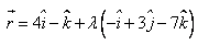. The Cartesian equation of the line is:

Solution:
QUESTION: 6

Let the coordinates of the given point A be (x1, y1, z1) and the direction ratios of the line be a, b, c. If the co-ordinates of any point P is (x, y, z), then the equation of the line in Cartesian form is:

Solution:
QUESTION: 7

Let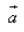be a position vector of A with respect to the origin O and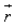be a position vector of an arbitrary point. The equation of line which passes through A and parallel to a vector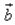is:

Solution:
QUESTION: 8

The Cartesian equation of the line passing through the points (-3, 1, 0) and (1, 2, 3) is:

Solution:
QUESTION: 9

The Cartesian equation of the line which passes through the origin and parallel to the line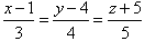, is given by

Solution:
QUESTION: 10

Find the Cartesian equation of the line that passes through the point (-3,-4,-2) and (3,4,2).

Solution: